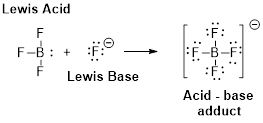Chapter 16, Problem 6PS

Chapter
Section
Textbook Problem

Write a balanced equation showing how the HPO 4 2 − ion of sodium hydrogen phosphate Na 2 HPO 4 can be a both conjugated acid and conjugated base.

Interpretation Introduction

Interpretation:

Balanced equation of HPO42- ion of sodium hydrogen phosphate (Na2HPO4) has to be written and the conjugated acid and conjugated base has to be shown.

Concept introduction:

Balanced reaction is a chemical reaction in which number of atoms for each element in the reaction and the total charge are same on both reactant side and the product side.

Steps in balancing the information

• Step 1: Write the unbalanced equation
• Step 2: Find the coeffienct to balance the equation.
• The coeffienct should be reduced to the smallest whole number

Bronsted –Lowry definitions:

A Bronsted –Lowry acid is a proton donor, it donates a hydrogen ion, H+

A Bronsted-Lowry base is a proton acceptor, it accepts a hydrogen ion H+Bronsted –Lowry conjugated acid-Base pairs:

When an acid is dissolved in water, the acid (HA) donates a proton to water to form a new acid (conjugated acid) and a new base (conjugated base).The pair of an Acid –Base differs by a proton called conjugated Acid-Base pair.

Explanation

Sodium hydrogen phosphate (Na2HPO4) dissociates into HPO42- and sodium ion.

Na2HPO4   2Na++ HPO42-

HPO42- accepts a proton from water molecule to form its conjugated acid.

The chemical reaction is as follows.

HPO42- + H2H2PO4       + OH                                         Conjugated

Still sussing out bartleby?

Check out a sample textbook solution.

See a sample solution

The Solution to Your Study Problems

Bartleby provides explanations to thousands of textbook problems written by our experts, many with advanced degrees!

Get Started

Find more solutions based on key concepts